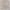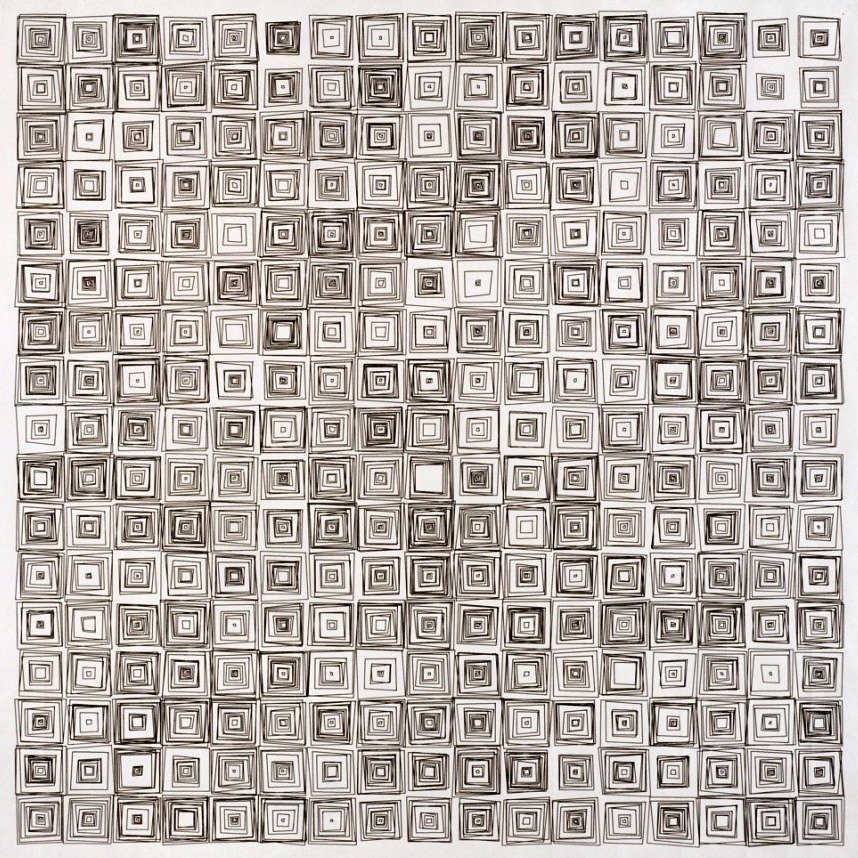# Replica analysis of Bayesian data clustering

We optimize Bayesian data clustering by mapping the problem to the statistical physics of a gas and calculating the lowest entropy state.

Journal of Physics A 53, 3 (2019)We use statistical mechanics to study model-based Bayesian data clustering. In this approach, each partition of the data into clusters is regarded as a microscopic system state, the negative data log-likelihood gives the energy of each state, and the data set realisation acts as disorder. Optimal clustering corresponds to the ground state of the system, and is hence obtained from the free energy via a low `temperature' limit. We assume that for large sample sizes the free energy density is self-averaging, and we use the replica method to compute the asymptotic free energy density. The main order parameter in the resulting (replica symmetric) theory, the distribution of the data over the clusters, satisfies a self-consistent equation which can be solved by a population dynamics algorithm. From this order parameter one computes the average free energy, and all relevant macroscopic characteristics of the problem. The theory describes numerical experiments perfectly, and gives a significant improvement over the mean-field theory that was used to study this model in past.#### Replica analysis of Bayesian data clustering

A. Mozeika, T. Coolen

Journal of Physics A

1 / 123 papers